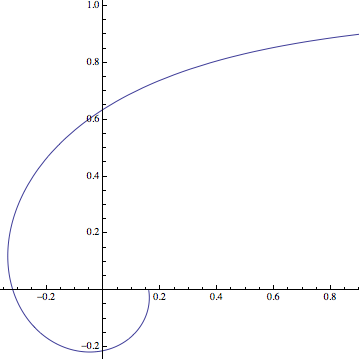# Arclength Of $r = 1/\theta$

Calculus Level 4

Consider the polar curve with equation $r = \dfrac1\theta$. What is the length of the arc traced by this curve as $\theta$ (measured in radians) varies from 1 to 2?A plot of the polar curve $r = \dfrac1\theta$.

×